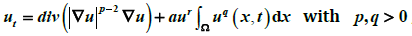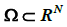# 带非局部源的p-Laplace发展方程解的熄灭Extinction for a Class of p-Laplace Evolution Equation with Nonlocal Sources

DOI: 10.12677/AAM.2014.32018, PDF, HTML, XML, 下载: 2,230  浏览: 7,325  科研立项经费支持

Abstract: In this article, the authors establish conditions for the extinction of nonlocal solutions, in finite time, of a class of evolution p-Laplace equationin a bounded domainwith N > 2 .

  Kalashnikov, A.S. (1974) The propagation of disturbances in problems of nonlinear heat conduction with absorption. USSR Computational Mathematics and Mathematical Physics, 14, 70-85.  Lair, A.V. (1993) Finite extinction time for solutions of nonlinear parabolic equations. Nonlinear Analysis: Theory, Methods & Applications, 21, 1-8.  Lair, A.V. and Oxley, M.E. (1994) Extinction in finite time for a nonlinear absorption diffusion equation. Journal of Mathematical Analysis and Applications, 182, 857-866.  Lair, A.V. and Oxley, M.E. (1994) Finite extinction time for a nonlinear parabolic Neumann boundary value problem. Applied Analysis, 55, 41-50.  顾永耕 (1994) 抛物型方程的解熄灭的充要条件. 数学学报, 37, 73-79.  Li, Y.X. and Wu, J.C. (2005) Extinction for fast diffusion equations with nonlinear sources. Electronic Journal of Dif- ferential Equations, 2005, 1-7.  Wang, M.X. and Wang, Y.M. (1996) Properties of positive solutions for non-local reaction-diffusion problems. Ma- thematical Methods in the Applied Sciences, 19, 1141-1156.  Lin, Z.G. and Liu, Y.R. (2004) Uniform blow up profiles for diffusion equations with nonlocal source and nonlocal boundary. Acta Mathematica Scientia, 24, 443-450.  陈玉娟 (2006) 非局部的退化抛物型方程组的解的爆破和整体存在性. 数学物理学报, 26A, 731-740.  Fang, Z.B. and Xu, X.H. (2012) Extinction behavior of solutions for the p-Laplacian equations with nonlocal sources. Nonlinear Analysis: Real World Applications, 13, 1780-1789.  Han, Y.Z. and Gao, W.J. (2011) Extinction for a fast diffusion equation with a nonlinear nonlocal source. Archiv der Mathematik, 97, 353-363.  Tian, Y. and Mu, C.L. (2008) Extinction and non-extinction for a p-Laplacian equation with nonlinear source. Nonlinear Analysis, 69, 2422-2431.